# Most important scientific and applied results

1. Analytical computer calculation of a volume integrals on areas of integration, specified by convex polyhedra with moving boundaries in n-dimensional space

2. Software and discrete-combinatorial methods for formulas analytical calculation describing the probability of error-free random dot images reading

3. A multidimensional generalization of the classic Catalan numbers

4. Software-algorithmic complex for Earth's surface area stereo reconstruction with satellite data obtained from low-orbit spacecraft

5. Programs and algorithms improving the spatial resolution of digital images

6. Algorithms for estimating the relative fragments displacement of digital images

7. Programs and algorithms for the optimal time detection and localization of impulse objects

Section 1. Analytical computer calculation of a volume integrals on areas of integration, specified by convex polyhedra with moving boundaries in n-dimensional space

Software package has been created for formulas analytical calculation defined by multidimensional integrals of the form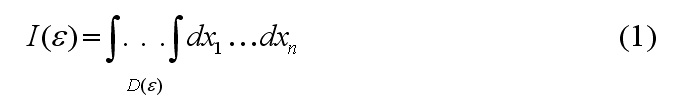where the domain of integrationis limited to a system of linear inequalitiesA software baseline algorithm is based on the idea the integral can be presented in the equivalent form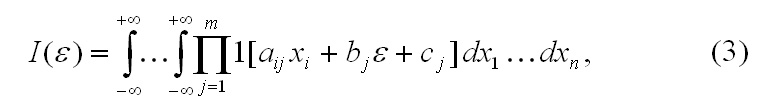when the indicator function of the set is brought under the integral sign, and the integration is extended to the whole space. Heaviside functions are factors in the integrand: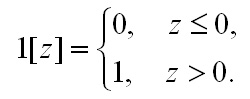Further n-dimensional integral (3) (using a cyclic identity)is converted to a set of repeated integrals with the set limits of integration. At the final stage there is a consistent integration of each of the repeated integrals (from the splitting of original integral (1)), and then combining the results obtained, taking into account the changes of free boundary parameter e.

Section 2. Software and discrete-combinatorial methods for formulas analytical calculation describing the probability of error-free random dot images readingPreviously unknown analytical expressions have been established and proven using software and algorithmic tools, based on the analytical calculation of multidimensional integral expressions on convex polyhedra (see. Section 1), and a new concept of generalized Catalan numbers (see. Section 3). Expressions describe the probability of error-free registration of random dot image coordinates in the case of limited number of threshold levels of scanning aperture.
There is probability formulas table for calculated using specialized analytical integration software systems table of that determine the particular problem solutions for fixed values n and k (k <n) up to n=14, it can be found here. Closed to the parameter n General form of analytical relations describing the reliability of the random dot images reading process, can be found here (in russian).

Section 3. A multidimensional generalization of the classic Catalan numbersThe concept of multidimensional generalized Catalan number has been introduced. For each of the proposed classical Catalan numbers extension its explicit analytical representation has been found. The new concept applied effectively in research related to reliability of random dot images reading. The proposed extension of the classical Catalan sequence (known since Leonhard Euler’s research), is a significant contribution to the development of enumerative combinatorics, and this is a useful tool in solving many theoretical and applied probability and combinatorial problems. In our studies, the need for classic Catalan numbers expansion appeared in solving problems related to the reliability of random dot images reading. Problems leading to generalized Catalan number have been formulated in an special word-linguistic form and together with the decisions can be found here.

Section 4. Software-algorithmic complex for Earth's surface area stereo reconstruction with satellite data obtained from low-orbit spacecraftA comprehensive approach was developed based on software support (through all stages) of the automatic recovery of the Earth surface’s 3d-relief from satellite flight’s data, requiring no operator intervention.
In this paper we propose efficient methods for finding conjugate points, estimation of main point’s unknown coordinates and rotation angles of the camera. An important feature of the developed algorithms is their high performance.
These algorithms are based on the joint-group parallel processing of several stereoscopic projection that makes the solution robust and significantly reduces the time it is received

Section 5. Programs and algorithms improving the spatial resolution of digital images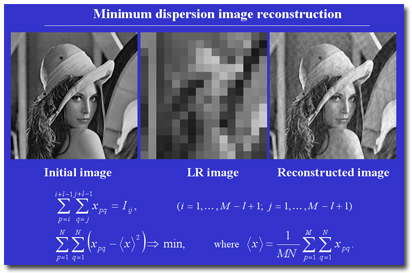Algorithms and software system created for obtaining super-resolution images from sequence of low-quality images obtained by controlled sub-pixel scan.
At the core of the implemented algorithms lies the method of minimum energy image searching. Algorithms running steadily on image edges (with almost no impact on restoration quality) and show acceptable results in the presence of additive noise.

Section 6. Algorithms for estimating the relative fragments displacement of digital imagesPrecise estimation algorithms for digital image’s fragments relative displacement was created based on bilinear interpolation of the original signal, independent interpolation for each variable, the signal’s series expansion and other methods. Best of the developed algorithms shows highly accurate alignment results (even with a significant level of noise), because they are based on statistical averaging of partial derivative signal’s sample values over the field

Section 7. Programs and algorithms for the optimal time detection and localization of impulse objectsOptimal algorithms have been created for localization of dot-pulse objects forming the random field and appearing at random time in the form of infinitely short delta-pulses generation. The optimal search algorithm must usually satisfy one of two conditions: either minimizing the total search effort required to detect an object, or maximizing the total probability of detection in the presence of a limited search efforts. Procedure of the search and localization of random dot-pulse objects uses the tunable-viewing-window receiver.

The optimal strategy for the one-step, multi-stage, cyclic and multi-search has been found.- Average time of localization of the object with the one-step search process.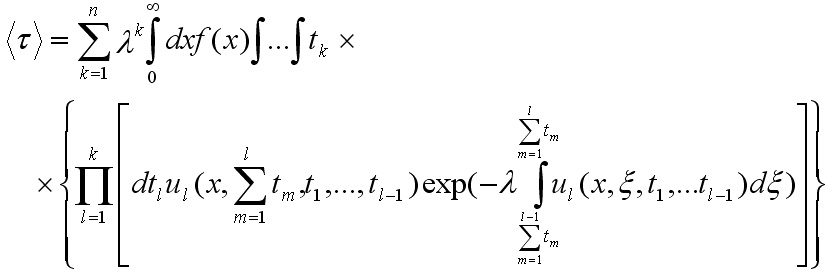Average time of localization of the object with a multi-stage search process.

The list of publications (Lab 12) can be found here.